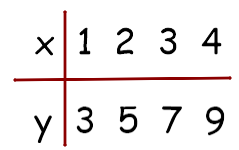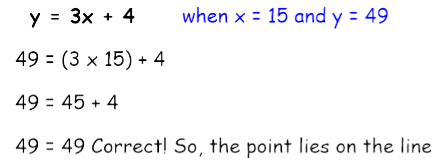# Investigate Straight Line Graphs

In this worksheet, students will explore straight line graphs furtherKey stage:  KS 3

Curriculum topic:   Algebra

Curriculum subtopic:   Use Linear Equations (Two Variables) and Gradients

Difficulty level:#### Worksheet Overview

A straight line graph is a sequence of numbers which go up or down by the gradient.

For example

3, 5, 7, 9, ......      has the rule  2n + 1 because it goes up in 2s and each term is 1 more than the 2 times table.

When we draw a straight line graph with a gradient of 2 and a y-intercept of 1 we write y = 2x + 1.

If we plot the table of values we find that sequence:Therefore, we can create our own coordinates if we have the straight line graph.

Where are we going with this? Let's look at a question to make it clearer.

Example

Does the point (15, 49) lie on the line y = 3x + 4?

Firstly, we look at y = 3x + 4 and we know that:

the gradient is 3 (it goes up in 3s)

the y-intercept is +4  (so, we know that (0, 4) is a point on the line)

If we take the point (15, 49) it means that when x = 15 then y = 49

If it lies on the line y = 3x + 4 we should be able to substitute these values in and the equation should be true!If the equation is not equal then the point does not lie on the line!

Let's try some of these questions.### What is EdPlace?

We're your National Curriculum aligned online education content provider helping each child succeed in English, maths and science from year 1 to GCSE. With an EdPlace account you’ll be able to track and measure progress, helping each child achieve their best. We build confidence and attainment by personalising each child’s learning at a level that suits them.

Get started